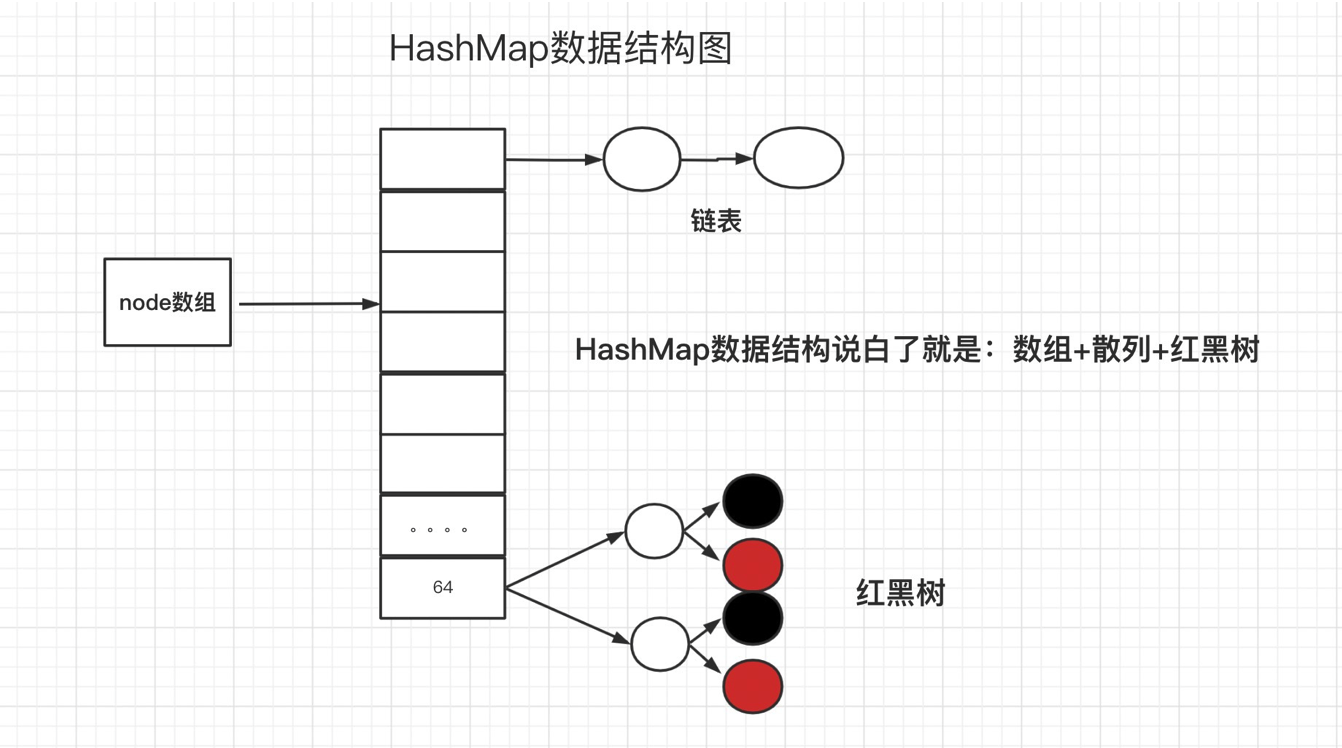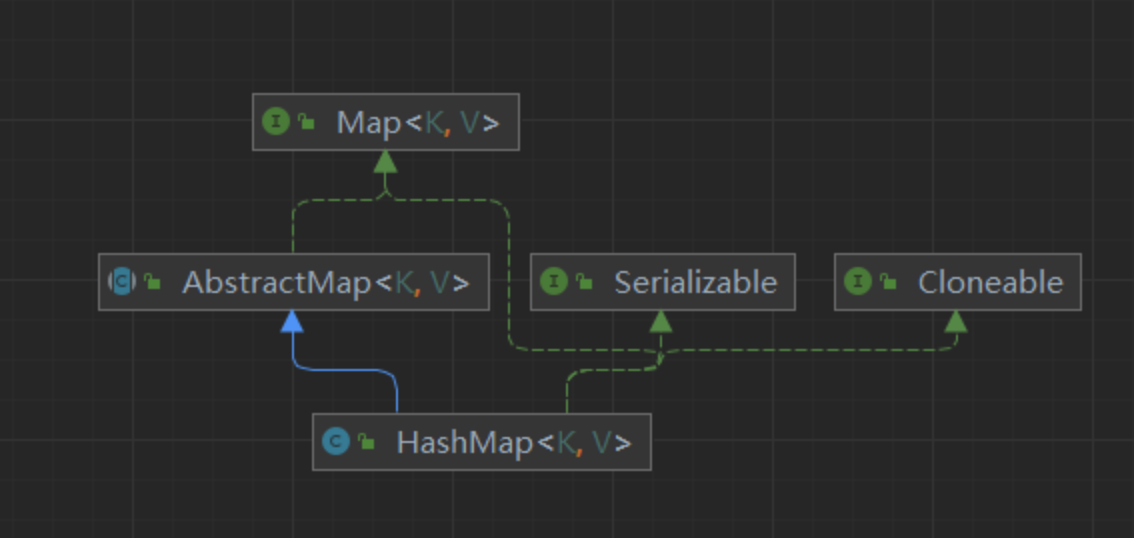## 一、HashMap是什么### HashMap继承树结构### HashMap特性总结

1.HashMap 是以 key-val对的方式来存储数据。

2.key不能重复，但是是值可以重复，允许使用null键和null值。
3.如果添加相同的key，则会覆盖原来的key-val等同于修改。
4.与HashSet一样，不保证映射的顺序，因为底层是以hash表的方式来存储的。
4.HashMap没有实现同步，因此是线程不安全的。

## 二、HashMap五个参数

### 1.初始容量

``````static final int DEFAULT_INITIAL_CAPACITY = 1 << 4; // aka 16
``````

### 2.负载因子

``````static final float DEFAULT_LOAD_FACTOR = 0.75f;
``````

### 3.最大容量

``````static final int MAXIMUM_CAPACITY = 1 << 30;
``````

### 4.树化阈值

``````static final int TREEIFY_THRESHOLD = 8;
``````

## 三、HashMap三个构造函数

``````public HashMap(int initialCapacity, float loadFactor) {
// 如果 initialCapacity（初始容量）小于0 抛出异常
if (initialCapacity < 0)
throw new IllegalArgumentException("Illegal initial capacity: " +
initialCapacity);
// 如果 initialCapacity（初始容量）大于 最大容量 把最大容量赋值给初始容量
// 在HashMap的官方文档中提到 初始容量不得大于 最大容量
if (initialCapacity > MAXIMUM_CAPACITY)
initialCapacity = MAXIMUM_CAPACITY;
// 负载因子 不得为空
throw new IllegalArgumentException("Illegal load factor: " +
this.threshold = tableSizeFor(initialCapacity);
}
``````

``````public HashMap() {
}
``````

``````public HashMap(Map<? extends K, ? extends V> m) {
putMapEntries(m, false);
}
``````

## 四、put方法源码解析

``````public V put(K key, V value) {
//  这里面 提供了一个hash()方法 这个方法的一个主要作用 就是计算出当前KEY的hash值

return putVal(hash(key), key, value, false, true);
}

// hash 寻址
static final int hash(Object key) {
int h;
// 将我们的hash值右移16位 减少hash冲突
// 即使高位为0，异或特性： 0 异或任何数 都为任何数它本身 ，那就是返回key.hashCode() ，不会影响它本身
return (key == null) ? 0 : (h = key.hashCode()) ^ (h >>> 16);
}
``````

put数据的幕后功臣putVal,在下面的过程中 我会 逐行为大家讲解 每一行代码的意思 以及作用

``````final V putVal(int hash, K key, V value, boolean onlyIfAbsent,
boolean evict) {
Node<K,V>[] tab; Node<K,V> p; int n, i;
// 判断我们当前table 是否为空 若为空 调用我们的resize()进行初始化
// 当然resize()也是我们的扩容方法
// 注 ： 频繁的resize() 势必会影响我们的HashMap的一个性能
if ((tab = table) == null || (n = tab.length) == 0)
n = (tab = resize()).length;
// 如果通过hash算出来的数组节点为null 就new一个节点  把该值存放进去
// 如果不为空 就走下面的 else 放入链表
if ((p = tab[i = (n - 1) & hash]) == null)
tab[i] = newNode(hash, key, value, null);
else {
// 链表操作
Node<K,V> e; K k;
// 判断 hash 算出的节点不为空
// 判断该节点与我们的key 是否相同 如果相同 就获取该节点
if (p.hash == hash &&
((k = p.key) == key || (key != null && key.equals(k))))
e = p;
// 如果不容 判断 该节点 是否 为 TreeNode  即 红黑树
// 否则
else if (p instanceof TreeNode)
// 如果为树 就以树的存储方式进行存储
e = ((TreeNode<K,V>)p).putTreeVal(this, tab, hash, key, value);
else {

for (int binCount = 0; ; ++binCount) {
if ((e = p.next) == null) {
// 如果是链表的，以key-value插到链表尾
// 插入尾部避免hash冲突造成死循环
p.next = newNode(hash, key, value, null);
// 节点 到达 阈值 进行树化
if (binCount >= TREEIFY_THRESHOLD - 1) // -1 for 1st
//  树化方法
// 并不一定 到达 阈值 就会树化 具体在 树化方法中 会对节点大小进行判
// 断
treeifyBin(tab, hash);
break;
}
// 判断 节点key 与我们要 插入的key 是否相等
if (e.hash == hash &&
((k = e.key) == key || (key != null && key.equals(k))))
// 相等 跳出循环 执行 替换操作
break;
p = e;
}
}
// 如果 e 不等于 null 就证明 我们即将插入的key 与 我们节点中的key相等
// 注： 这里也就是 hashMap 中 不管插入多少 都会 用新值 替换旧值得 关键代码
if (e != null) { // existing mapping for key
// 记录我们旧的value 值
V oldValue = e.value;
if (!onlyIfAbsent || oldValue == null)
// 新的vale值 替换
e.value = value;
// 回调
afterNodeAccess(e);
// 返回旧的值
return oldValue;
}
}
++modCount;
//  实际大小 大于 阈值 就扩容
if (++size > threshold)
resize();
afterNodeInsertion(evict);
return null;
}
``````

1.首先获取Node数组table对象和长度，若table为null或长度为0，则调用resize()扩容方法获取table最新对象，并通过此对象获取长度大小

2.判定数组中指定索引下的节点是否为Null，若为Null 则new出一个单向链表赋给table中索引下的这个节点

3.若判定不为Null,我们的判断再做分支

3.1 首先对hash和key进行匹配,若判定成功直接赋予e

3.2 若匹配判定失败,则进行类型匹配是否为TreeNode 若判定成功则在红黑树中查找符合条件的节点并将其回传赋给e

3.3 若以上判定全部失败则进行最后操作,向单向链表中添加数据若单向链表的长度大于等于8,则可能将其转为红黑树保存，记录下一个节点,对e进行判定若成功则返回旧值

4.最后判定数组大小需不需要扩容

## 五、treeifyBin 树化方法

``````final void treeifyBin(Node<K,V>[] tab, int hash) {
int n, index; Node<K,V> e;
// 判断数组 是否为空 以及 容量 是否大于 我们的 阈值容量 MIN_TREEIFY_CAPACITY=64
if (tab == null || (n = tab.length) < MIN_TREEIFY_CAPACITY)
// 扩容方法
resize();
// 否则 就 树化
else if ((e = tab[index = (n - 1) & hash]) != null) {
TreeNode<K,V> hd = null, tl = null;
do {
//	 node 转为 TreeNode
TreeNode<K,V> p = replacementTreeNode(e, null);
if (tl == null)
hd = p;
else {
p.prev = tl;
tl.next = p;
}
tl = p;
} while ((e = e.next) != null);
if ((tab[index] = hd) != null)
hd.treeify(tab);
}
}
``````

## 六、resize 扩容方法

``````//重新设置table大小/扩容 并返回扩容的Node数组即HashMap的最新数据
final Node<K,V>[] resize() {
Node<K,V>[] oldTab = table; //table赋予oldTab作为扩充前的table数据
int oldCap = (oldTab == null) ? 0 : oldTab.length;
int oldThr = threshold;
int newCap, newThr = 0;
if (oldCap > 0) {
//判定数组是否已达到极限大小，若判定成功将不再扩容，直接将老表返回
if (oldCap >= MAXIMUM_CAPACITY) {
threshold = Integer.MAX_VALUE;
return oldTab;
}
//若新表大小(oldCap*2)小于数组极限大小 并且 老表大于等于数组初始化大小
else if ((newCap = oldCap << 1) < MAXIMUM_CAPACITY &&
oldCap >= DEFAULT_INITIAL_CAPACITY)
//旧数组大小oldThr 经二进制运算向左位移1个位置 即 oldThr*2当作新数组的大小
newThr = oldThr << 1; // double threshold
}
//若老表中下次扩容大小oldThr大于0
else if (oldThr > 0)
newCap = oldThr;  //将oldThr赋予控制新表大小的newCap
else { //若其他情况则将获取初始默认大小
newCap = DEFAULT_INITIAL_CAPACITY;
}
//若新表的下表下一次扩容大小为0
if (newThr == 0) {
float ft = (float)newCap * loadFactor;  //通过新表大小*负载因子获取
newThr = (newCap < MAXIMUM_CAPACITY && ft < (float)MAXIMUM_CAPACITY ?
(int)ft : Integer.MAX_VALUE);
}
threshold = newThr; //下次扩容的大小
@SuppressWarnings({"rawtypes","unchecked"})
Node<K,V>[] newTab = (Node<K,V>[])new Node[newCap];
table = newTab; //将当前表赋予table
if (oldTab != null) { //若oldTab中有值需要通过循环将oldTab中的值保存到新表中
for (int j = 0; j < oldCap; ++j) {
Node<K,V> e;
if ((e = oldTab[j]) != null) {//获取老表中第j个元素 赋予e
oldTab[j] = null; //并将老表中的元素数据置Null
if (e.next == null) //若此判定成立 则代表e的下面没有节点了
newTab[e.hash & (newCap - 1)] = e; //将e直接存于新表的指定位置
else if (e instanceof TreeNode)  //若e是TreeNode类型
//分割树，将新表和旧表分割成两个树，并判断索引处节点的长度是否需要转换成红黑树放入新表存储
((TreeNode<K,V>)e).split(this, newTab, j, oldCap);
else { // preserve order
Node<K,V> loHead = null, loTail = null; //存储与旧索引的相同的节点
Node<K,V> hiHead = null, hiTail = null; //存储与新索引相同的节点
Node<K,V> next;
//通过Do循环 获取新旧索引的节点
do {
next = e.next;
if ((e.hash & oldCap) == 0) {
if (loTail == null)
else
loTail.next = e;
loTail = e;
}
else {
if (hiTail == null)
else
hiTail.next = e;
hiTail = e;
}
} while ((e = next) != null);
//通过判定将旧数据和新数据存储到新表指定的位置
if (loTail != null) {
loTail.next = null;
}
if (hiTail != null) {
hiTail.next = null;
}
}
}
}
}
//返回新表
return newTab;
}
``````

1.判定数组是否已达到极限大小，若判定成功将不再扩容，直接将老表返回

2.若新表大小(oldCap2)小于数组极限大小&老表大于等于数组初始化大小 判定成功则 旧数组大小oldThr 经二进制运算向左位移1个位置 即 oldThr2当作新数组的大小

2.1. 若的判定不成功，则继续判定 oldThr （代表 老表的下一次扩容量）大于0，若判定成功 则将oldThr赋给newCap作为新表 的容量

2.2 若  和[2.1]判定都失败,则走默认赋值 代表 表为初次创建

3.确定下一次表的扩容量, 将新表赋予当前表

4.通过for循环将老表中数值存入扩容后的新表中

4.1 获取旧表中指定索引下的Node对象 赋予e 并将旧表中的索引位置数据置空

4.2 若e的下面没有其他节点则将e直接赋到新表中的索引位置

4.3 若e的类型为TreeNode红黑树类型

4.3.1 分割树，将新表和旧表分割成两个树，并判断索引处节点的长度是否需要转换成红黑树放入新表存储

4.3.2 通过Do循环 不断获取新旧索引的节点

4.3.3 通过判定将旧数据和新数据存储到新表指定的位置

## 七、面试问题

### 2、说说你对红黑树的见解？

1、每个节点非红即黑

2、根节点总是黑色的

3、如果节点是红色的，则它的子节点必须是黑色的（反之不一定）

4、每个叶子节点都是黑色的空节点（NIL节点）

5、从根节点到叶节点或空子节点的每条路径，必须包含相同数目的黑色节点（即相同的黑色高度）